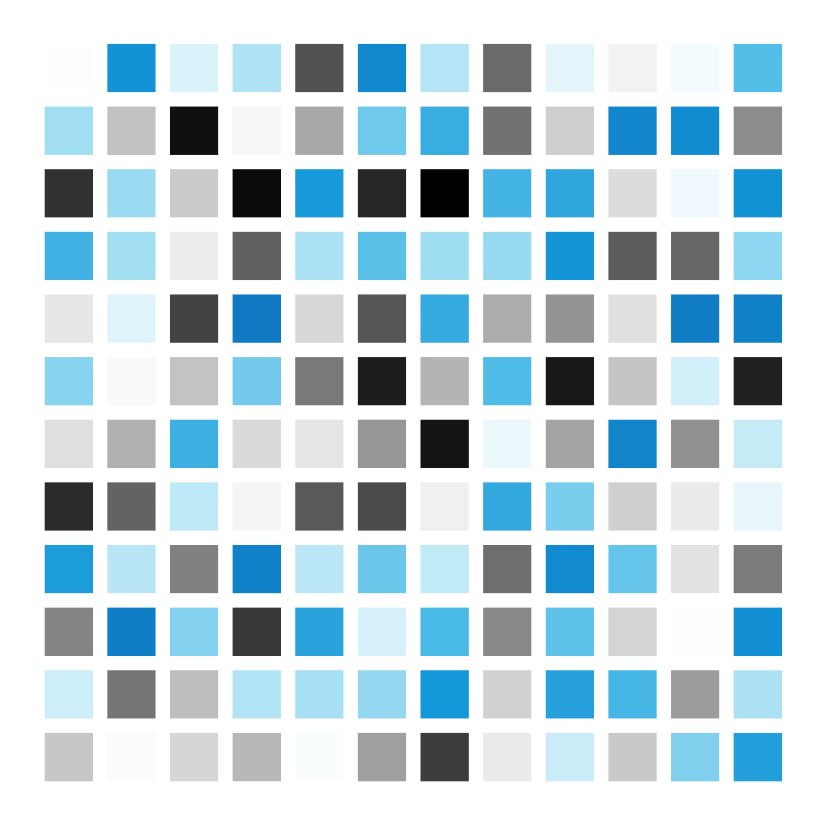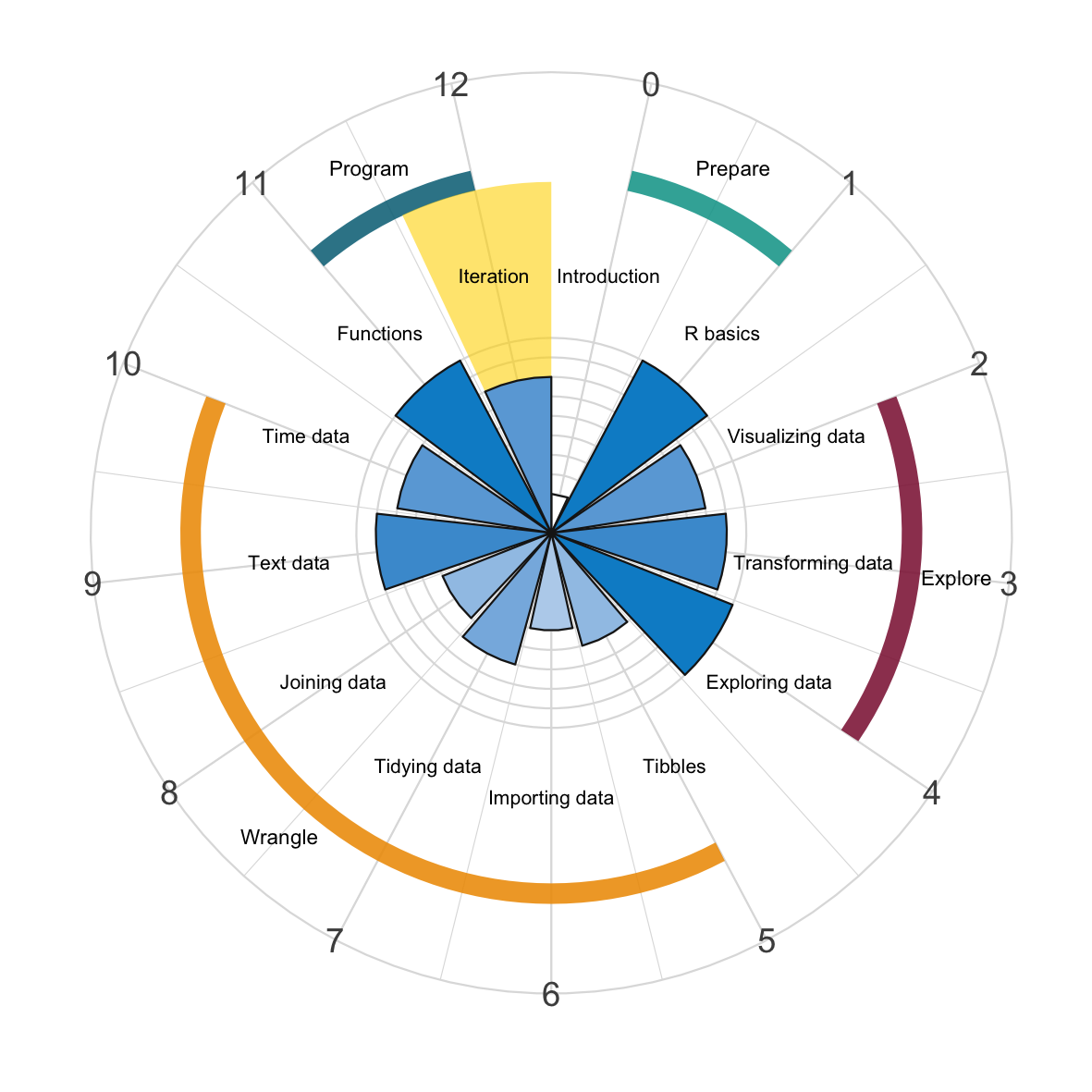# Chapter 12 IterationThe notion of “iteration” is an essential concept of computer programming. Basically, iteration is a method for tackling tasks that have the general form “for each $$X$$, do $$Y$$”. Thus, implies solving some task $$Y$$ repeatedly ($$X$$ times). Whenever a procedure or process requires repetitions, computers really begin to shine.Figure 12.1: Iteration with for loops, apply(), or the map() functions of purrr.

The traditional way to handle iteration is by repeating code in loops. When the number of required iterations is known in advance, we can use for loops to repeat some code for the desired number of times. In cases for which the number of iterations cannot be known beforehand, a while loop provides a general way for repeatedly executing some code.

As R is a functional programming language, it provides additional ways of applying functions repeatedly to data structures. As this book focuses on the essentials, we will primarily discuss for and while loops here, and only briefly touch upon the base R apply and the map family of functions of the purrr package .

Together with our previous chapter on Functions (see Chapter 11), this chapter concludes our brief part on computer programming.

### References

Henry, L., & Wickham, H. (2023). purrr: Functional programming tools. Retrieved from https://purrr.tidyverse.org
R Core Team. (2023). R base: A language and environment for statistical computing. Retrieved from https://www.R-project.org